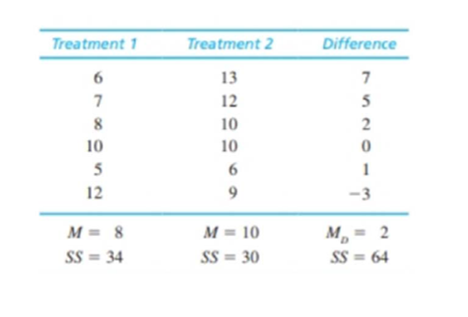Chapter 11, Problem 19PEssentials of Statistics for the B...

8th Edition
Frederick J Gravetter + 1 other
ISBN: 9781133956570

Solutions

Chapter
SectionEssentials of Statistics for the B...

8th Edition
Frederick J Gravetter + 1 other
ISBN: 9781133956570
Textbook Problem

Problem 18 shows that removing individual differences can substantially reduce variance and lower the standard error. However, this benefit only occurs if the individual differences are consistent across treatment conditions. In problem 18, for example, the first two participants (top two rows) consistently had the highest scores in both treatment conditions. Similarly, the last two participants consistently had the lowest scores in both treatments. To construct the following data, we started with the scores in problem 18 and scrambled the scores in treatment 1 to eliminate the consistency of the individual differences. a. Assume that the data are from an independent-measures study using two separate samples, each with n = 6 participants. Compute the pooled variance and the estimated standard error for the mean difference. b. Now assume that the data are from a repeated measures study using the same sample of n = 6 participants in both treatment conditions. Compute the variance for the sample of difference scores and the estimated standard error for the mean difference. (This time you should find that removing the individual differences does not reduce the variance or the standard error.)a.

To determine

To find: The value of pooled variance and standard error.

Explanation

Given info:

The independent-measures study with scores of n=6 participants is used and samples means of the two treatments are 8 and 10 while SS values are 34 and 30 respectively. Also, the means for  difference is 2 while SS value is 64

Calculation:

The pooled variance, sp2, is calculated using formula:

sp2=SS1+SS2df1+df2

Substitute 34 for SS1, 30 for SS2 and 5 for both df1 and df2 in the above formula

sp2=34+305+5=6

b.

To determine

To find: The value of variance for the mean difference and standard error for the mean difference.

Still sussing out bartleby?

Check out a sample textbook solution.

See a sample solution

The Solution to Your Study Problems

Bartleby provides explanations to thousands of textbook problems written by our experts, many with advanced degrees!

Get Started

Finding a Limit In Exercises 21-24, find the limit. limx0cothx

Calculus: Early Transcendental Functions (MindTap Course List)

In Exercises 1124, find the indicated limits, if they exist. 14. limx3x3x+4

Applied Calculus for the Managerial, Life, and Social Sciences: A Brief Approach

In Exercises 1728, use the logarithm identities to obtain the missing quantity.

Finite Mathematics and Applied Calculus (MindTap Course List)

True or False: The function y = f(x) graphed at the right could be a solution to y = x + y.

Study Guide for Stewart's Single Variable Calculus: Early Transcendentals, 8th

Using a binomial series, the Maclaurin series for is:

Study Guide for Stewart's Multivariable Calculus, 8th